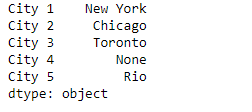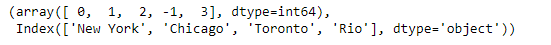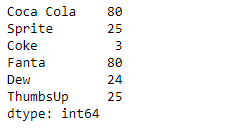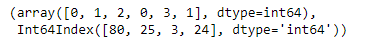Related Articles

# Python | Pandas Series.factorize()

• Last Updated : 13 Feb, 2019

Pandas series is a One-dimensional ndarray with axis labels. The labels need not be unique but must be a hashable type. The object supports both integer- and label-based indexing and provides a host of methods for performing operations involving the index.

Pandas` Series.factorize()` function encode the object as an enumerated type or categorical variable. This method is useful for obtaining a numeric representation of an array when all that matters is identifying distinct values.

Syntax: Series.factorize(sort=False, na_sentinel=-1)

Parameter :
sort : Sort uniques and shuffle labels to maintain the relationship.

Returns :
labels : ndarray
uniques : ndarray, Index, or Categorical

Example #1: Use `Series.factorize()` function to encode the underlying data of the given series object.

 `# importing pandas as pd``import` `pandas as pd`` ` `# Creating the Series``sr ``=` `pd.Series([``'New York'``, ``'Chicago'``, ``'Toronto'``, ``None``, ``'Rio'``])`` ` `# Create the Index``sr.index ``=` `[``'City 1'``, ``'City 2'``, ``'City 3'``, ``'City 4'``, ``'City 5'``] `` ` `# set the index``sr.index ``=` `index_`` ` `# Print the series``print``(sr)`

Output :Now we will use `Series.factorize()` function to encode the underlying data of the given series object.

 `# encode the values``result ``=` `sr.factorize()`` ` `# Print the result``print``(result)`

Output :As we can see in the output, the `Series.factorize()` function has successfully encoded the underlying data of the given series object. Notice missing values has been assigned a code of -1.

Example #2 : Use `Series.factorize()` function to encode the underlying data of the given series object.

 `# importing pandas as pd``import` `pandas as pd`` ` `# Creating the Series``sr ``=` `pd.Series([``80``, ``25``, ``3``, ``80``, ``24``, ``25``])`` ` `# Create the Index``index_ ``=` `[``'Coca Cola'``, ``'Sprite'``, ``'Coke'``, ``'Fanta'``, ``'Dew'``, ``'ThumbsUp'``]`` ` `# set the index``sr.index ``=` `index_`` ` `# Print the series``print``(sr)`

Output :Now we will use `Series.factorize()` function to encode the underlying data of the given series object.

 `# encode the values``result ``=` `sr.factorize()`` ` `# Print the result``print``(result)`

Output :As we can see in the output, the `Series.factorize()` function has successfully encoded the underlying data of the given series object.

Attention geek! Strengthen your foundations with the Python Programming Foundation Course and learn the basics.

To begin with, your interview preparations Enhance your Data Structures concepts with the Python DS Course. And to begin with your Machine Learning Journey, join the Machine Learning – Basic Level Course

My Personal Notes arrow_drop_up